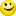# Multifunction DAQ

cancel
Showing results for
Did you mean:

## Calculating deviation between the rising edge and falling edge of the output signal of the Sensor

Hello,

I am  measuring the analog output signal (rectangular o/p) of the sensor using DAQ. I want to calculate the maximum deviation between rising edge and the falling edge of output signal. I tried to explain my ideas in the attached picture. The graph in the picture shows output waveform of my sensor and the below pic shows what I want to do. I have taken the mirror image of the falling edge and kept in parallel with rising edge to calculate deviation ( just to explain my task.) I want to program it in labview.

Does anyone have ideas or suggestions to do it?

regards,

Swap

Message 1 of 6
(3,668 Views)

## Re: Calculating deviation between the rising edge and falling edge of the output signal of the Sensor

Dear Swap,

This is a very unusual Task because a normal rectangular Signal should be 100% symmetrical and so the deviation between rising edge and the falling edge should be 0.

So for better understanding I would ask you to explain you application to me and why you would like to measure the deviation between rising edge and the falling edge which should normally be 0.

Kind Regards,

SG3 | Applications Engineering | National Instruments | NIG |

Message 2 of 6
(3,634 Views)

## Re: Calculating deviation between the rising edge and falling edge of the output signal of the Sensor

Hi SG3,

You are right. I accidentally mentioned rectangular signal it's sinusoidal signal. sorry for the mistake. Actually I am getting this sinusoidal signal from steering angle sensor on which I am doing test (Lifecycle test) and I want test that the sensor output is perfect sinwave (i.e. no deviation). I want to calculate the deviation between the rising edge and falling edge (ofcourse it normally should be be zero). I want to know that is there any solution for it.

How can I do it ?

I hope I made my point clear to you.

regards,

Swap

Message 3 of 6
(3,628 Views)

## Re: Calculating deviation between the rising edge and falling edge of the output signal of the Sensor

Dear Swap,

Yes I got your problem and have a possible solution for you.

LabVIEW provides lot of analyses VIs which you can use to solve this problem but I would recommend you not to mirror the negative edge and then to measure the derivation.

Instead of this I would recommend you to compare the slope and the transition duration of the falling and rising edge which the "Transition Measurements 1 chan.vi" calculates you.

I think this both metrics should be enough to say if the rising and falling edge are exactly the same or and if not how they differ.

Here is a Screenshot of this short analyses and you will find the VI in the attachments of my post.Kind Regards,

SG3 | Applications Engineering | National Instruments | NIG |

Message 4 of 6
(3,597 Views)

## Re: Calculating deviation between the rising edge and falling edge of the output signal of the Sensor

HI SG3,

Thank you for the reply. I will implement it in my programm and let's see if it works fine for me....regards,

Swap

Message 5 of 6
(3,556 Views)

## Re: Calculating deviation between the rising edge and falling edge of the output signal of the Sensor

I would call deviations in the falling to rising edge of a sinus a change in frequency 🙂  or jitter .....

If you have a nice sine, stable for about 10 periodes try the tone detection vi or extract single tone information.vi

the nice thing about extract single tone information is, that you can export the residual signal 🙂

If you want to test the sensor you can do some more stuff if you have a reverece signal .... I asume you have a incremental reference... take that as the sample clock.

store a reference trace, calc mean traces, deviations of the mean and start trace......

Greetings from Germany
Henrik

LV since v3.1

“ground” is a convenient fantasy

'˙˙˙˙uıɐƃɐ lɐıp puɐ °06 ǝuoɥd ɹnoʎ uɹnʇ ǝsɐǝld 'ʎɹɐuıƃɐɯı sı pǝlɐıp ǝʌɐɥ noʎ ɹǝqɯnu ǝɥʇ'

Message 6 of 6
(3,553 Views)